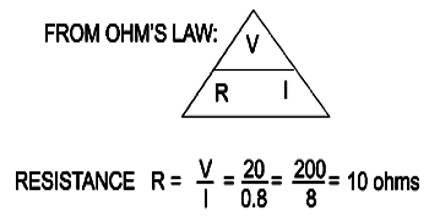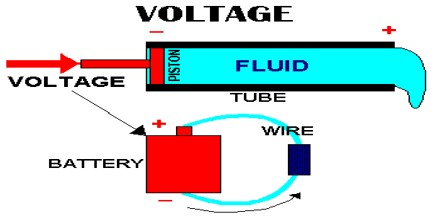About Voltage

Definition

Voltage is the electromotive force or the electrical potential difference between two points in a circuit expressed in volts. It is the pressure from an electrical circuit’s power source that pushes charged electrons (current) through a conducting loop, enabling them to do work such as illuminating a light. The voltage between two points is equal to the work done per unit of charge against a static electric field to move the test charge between two points. This is measured in units of volts.In brief, voltage = pressure, and it is measured in volts (V). The term recognizes Italian physicist Alessandro Volta (1745-1827), inventor of the voltaic pile—the forerunner of today’s household battery.

In electricity’s early days, voltage was known as electromotive force (emf). This is why in equations such as Ohm’s Law; voltage is represented by the symbol E.

Voltage can be direct or alternating. A direct voltage maintains the same polarity at all times. In an alternating voltage, the polarity reverses direction periodically. The number of complete cycles per second is the frequency, which is measured in hertz (one cycle per second), kilohertz, megahertz, gigahertz, or terahertz.

A voltmeter can be used to measure the voltage (or potential difference) between two points in a system; often a common reference potential such as the ground of the system is used as one of the points. A voltage may represent either a source of energy (electromotive force) or lost, used, or stored energy.

Units of Voltage

The SI unit of voltage is the volt, such that 1 volt = 1 joule/coulomb. It is represented by V. The volt is named after Italian physicist Alessandro Volta who invented a chemical battery.

This means that one coulomb of charge will gain one joule of potential energy when it is moved between two locations where the electric potential difference is one volt. For a voltage of 12 between two locations, one coulomb of charge will gain 12 joules of potential energy.A six-volt battery has a potential for one coulomb of charge to gain six joules of potential energy between two locations. A nine-volt battery has a potential for one coulomb of charge to gain nine joules of potential energy.

Ohm’s Law says voltage equals current times resistance. V = I * R. If you have a 12-volt battery but your resistance is two ohms, your current will be six amps. If the resistance were one ohm, your current would be 12 amps.

Applications of Voltage

Voltage is intimately connected with electrical energy. There exists AC and DC voltage regulators but by far the most common is the DC voltage regulator.  In DC regulators almost every internal combustion engine has a device called a voltage regulator which help tame the alternator output, but by far the widest use is DC voltage regulators for electronics circuits.The voltage between A and C is the sum of the voltage between A and B and the voltage between B and C. The various voltages in a circuit can be computed using Kirchhoff’s circuit laws.

All appliances are rated for an specific voltage (either DC or AC) then generators give a rather stable voltage (controlled on the exciter) but transmission lines could not provide same voltage all along then the AC might need some stabilizer to keep AC within range, voltage regulators is applied to DC supplies as well than on AC supplies and its purpose is to keep voltage within appliances range in spite of line voltage variation, either from generation or due to loads change producing serial resistivity drops, any device charger should have some kind of regulation and most of new appliances (TV, radio) do have some internal regulator allowing a wide range of input voltage.Common voltages supplied by power companies to consumers are 110 to 120 volts (AC) and 220 to 240 volts (AC). The voltage in electric power transmission lines used to distribute electricity from power stations can be several hundred times greater than consumer voltages, typically 110 to 1200 kV (AC).

Reference: techtarget.comthoughtco.comlifewire.com, wikipedia.